# PowerPoint Math Equations

Contents
[ ]

## Overview

In PowerPoint, it is possible to write a math equation or formula and display it in the presentation. To do that, various mathematical symbols are represented in PowerPoint and can be added to the text or equation. For that, the math equations constructor is used in PowerPoint, which helps to create complex formulas like:

• Math Fraction
• Math Function
• Limits and log functions
• N-ary operations
• Matrix
• Large operators
• Sin, cos functions

To add a mathematical equation in PowerPoint, the Insert -> Equation menu is used: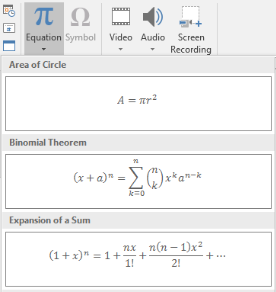This will create a mathematical text in XML that can be displayed in PowerPoint as following: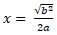PowerPoint supports plenty of mathematical symbols to create math equations. However, creating complicated math equations in PowerPoint often does not bring a good and professional-looking result. Users, who need to create mathematical presentations frequently, resort to the use of third-party solutions to create good-looking math formulas.

Using Aspose.Slide API, you can work with math equations in the PowerPoint presentations programmatically in C#. Create new math expressions or edit previously created ones. The export of mathematical structures into images is also partially supported.

## How to Create a Mathematical Equation

Mathematical elements are used for building any mathematical constructions with any level of nesting. A linear collection of mathematical elements forms a mathematical block represented by the MathBlock class. MathBlock class essentially is a separated mathematical expression, formula, or equation. MathPortion is a mathematical portion, used to hold mathematical text (do not mix with Portion). MathParagraph allows manipulating a set of math blocks. The abovementioned classes are the key to work with PowerPoint math equations via Aspose.Slides API.

Let’s see how we can create the following mathematical equation via Aspose.Slides API: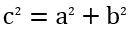To add a mathematical expression on the slide, first, add a shape that will contain the mathematical text:

``````
using (Presentation pres = new Presentation())

{

var mathShape = pres.Slides.Shapes.AddMathShape(0, 0, 720, 150);

}

``````

After creating, the shape will already contain one paragraph with a mathematical portion by default. The MathPortion class is a portion that contains a mathematical text inside. To access mathematical content inside MathPortion, refer to the MathParagraph variable:

``````
var mathParagraph = (mathShape.TextFrame.Paragraphs.Portions as MathPortion).MathParagraph;

``````

The MathParagraph class allows to read, add, edit and delete math blocks (MathBlock), that consist of a combination of mathematical elements. For example, create a fraction and place it in the presentation:

``````
var fraction = new MathematicalText("x").Divide("y");

``````

Each mathematical element is represented by some class that implements the IMathElement interface. This interface provides a lot of methods for easily creating mathematical expressions. You can create a fairly complex mathematical expression with a single line of code. For example, the Pythagorean theorem would look like this:

``````
var mathBlock = new MathematicalText("c")

.SetSuperscript("2")

.Join("=")

.Join(new MathematicalText("a").SetSuperscript("2"))

.Join("+")

.Join(new MathematicalText("b").SetSuperscript("2"));

``````

Operations of the interface IMathElement are implemented in any type of element, including the MathBlock.

The full source code sample:

``````
using (Presentation pres = new Presentation())

{

IAutoShape mathShape = pres.Slides.Shapes.AddMathShape(0, 0, 720, 150);

var mathParagraph = (mathShape.TextFrame.Paragraphs.Portions as MathPortion).MathParagraph;

var fraction = new MathematicalText("x").Divide("y");

var mathBlock = new MathematicalText("c")

.SetSuperscript("2")

.Join("=")

.Join(new MathematicalText("a").SetSuperscript("2"))

.Join("+")

.Join(new MathematicalText("b").SetSuperscript("2"));

pres.Save("math.pptx", SaveFormat.Pptx);

}

``````

## Mathematical Element Types

Mathematical expressions are formed from sequences of mathematical elements. The sequence of mathematical elements is represented by a mathematical block, and arguments of mathematical elements form a tree-like nesting.

There are a lot of mathematical element types that can be used to construct a mathematical block. Each of these elements can be included (aggregated) in another element. That is, elements are actually containers for others, forming a tree-like structure. The simplest type of element that does not contain other elements of the mathematical text.

Each type of math element implements the IMathElement interface, allowing the use of the common set of math operations on different types of math elements.

### MathematicalText class

The MathematicalText class represents a mathematical text - the underlying element of all mathematical constructions. Mathematical text may represent operands and operators, variables, and any other linear text.

Example: 𝑎=𝑏+𝑐

### MathFraction class

MathFraction class specifies the fraction object, consisting of a numerator and denominator separated by a fraction bar. The fraction bar can be horizontal or diagonal, depending on the fraction properties. The fraction object is also used to represent the stack function, which places one element above another, with no fraction bar.

Example: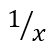MathRadical class specifies the radical function (mathematical root), consisting of a base, and an optional degree.

Example: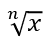### MathFunction class

MathFunction class specifies a function of an argument. Contains properties: Name - function name and Base - function argument.

Example: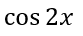### MathNaryOperator class

MathNaryOperator class specifies an N-ary mathematical object, such as Summation and Integral. It consists of an operator, a base (or operand), and optional upper and lower limits. Examples of N-ary operators are Summation, Union, Intersection, Integral.

This class does not include simple operators such as addition, subtraction, and so on. They are represented by a single text element - MathematicalText.

Example: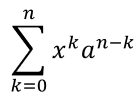### MathLimit class

MathLimit class creates the upper or lower limit. It specifies the limit object, consisting of text on the baseline and reduced-size text immediately above or below it. This element does not include the word “lim", but allows you to place text at the top or at the bottom of the expression. So, the expression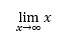is created using a combination of MathFunction and MathLimit elements this way:

``````
var funcName = new MathLimit(new MathematicalText("lim"), new MathematicalText("𝑥→∞"));

var mathFunc = new MathFunction(funcName, new MathematicalText("𝑥"));

``````

### MathSubscriptElement, MathSuperscriptElement, MathRightSubSuperscriptElement, MathLeftSubSuperscriptElement classes

The following classes specify a lower index or an upper index. You can set subscript and superscript at the same time on the left or on the right side of an argument, but single subscript or superscript is supported on the right side only. The MathSubscriptElement can also be used to set the mathematical degree of a number.

Example: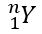### MathMatrix class

MathMatrix class specifies the Matrix object, consisting of child elements laid out in one or more rows and columns. It is important to note that matrixes do not have built-in delimiters. To place the matrix in the brackets you should use the delimiter object - IMathDelimiter. Null arguments can be used to create gaps in matrices.

Example: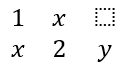### MathArray class

MathArray class specifies a vertical array of equations or any mathematical objects.

Example: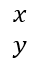### Formatting Mathematical Elements

• MathBorderBox class: draws a rectangular or some other border around the IMathElement.

Example: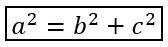• MathBox class: specifies the logical boxing (packaging) of the mathematical element. For example, a boxed object can serve as an operator emulator with or without an alignment point, serve as a line breakpoint, or be grouped such as not to allow line breaks within. For example, the “==” operator should be boxed to prevent line breaks.

• MathDelimiter class: specifies the delimiter object, consisting of opening and closing characters (such as parentheses, braces, brackets, and vertical bars), and one or more mathematical elements inside, separated by a specified character. Examples: (𝑥2); [𝑥2|𝑦2].

Example: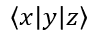• MathAccent class: specifies the accent function, consisting of a base and a combining diacritical mark.

Example: 𝑎́.

• MathBar class: specifies the bar function, consisting of a base argument and an overbar or underbar.

Example: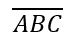• MathGroupingCharacter class: specifies a grouping symbol above or below an expression, usually to highlight the relationships between elements.

Example: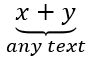## Mathematical Operations

Each mathematical element and mathematical expression (via MathBlock) implements the IMathElement interface. It allows you to use operations on the existing structure and form more complex mathematical expressions. All operations have two parameter sets: either IMathElement or string as arguments. Instances of the MathematicalText class are implicitly created from specified strings when string arguments are used. Math operations available in Aspose.Slides are listed below.

### Join method

Joins a mathematical element and forms a mathematical block. For example:

``````
IMathElement element1 = new MathematicalText("x");

IMathElement element2 = new MathematicalText("y");

IMathBlock block = element1.Join(element2);

``````

### Divide method

Creates a fraction of the specified type with this numerator and specified denominator. For example:

``````
IMathElement numerator = new MathematicalText("x");

IMathFraction fraction = numerator.Divide("y", MathFractionTypes.Linear);

``````

### Enclose method

Encloses the element in specified characters such as parenthesis or another character as framing.

``````
/// <summary>

/// Encloses a math element in parenthesis

/// </summary>

IMathDelimiter Enclose();

/// <summary>

/// Encloses this element in specified characters such as parenthesis or another characters as framing

/// </summary>

IMathDelimiter Enclose(char beginningCharacter, char endingCharacter);

``````

For example:

``````
IMathDelimiter delimiter = new MathematicalText("x"). Enclose('[', ']');

IMathDelimiter delimiter2 = new MathematicalText("elem1").Join("elem2").Enclose();

``````

### Function method

Takes a function of an argument using the current object as the function name.

``````
/// <summary>

/// Takes a function of an argument using this instance as the function name

/// </summary>

/// <param name="functionArgument">An argument of the function</param>

IMathFunction Function(IMathElement functionArgument);

IMathFunction Function(string functionArgument);

``````

For example:

``````
IMathFunction func = new MathematicalText("sin").Function("x");

``````

### AsArgumentOfFunction method

Takes the specified function using the current instance as the argument. You can:

For example:

``````
var funcName = new MathLimit(new MathematicalText("lim"), new MathematicalText("𝑛→∞"));

var func1 = new MathematicalText("2x").AsArgumentOfFunction(funcName);

var func2 = new MathematicalText("x").AsArgumentOfFunction("sin");

var func3 = new MathematicalText("x").AsArgumentOfFunction(MathFunctionsOfOneArgument.Sin);

var func4 = new MathematicalText("x").AsArgumentOfFunction(MathFunctionsOfTwoArguments.Log, "3")

``````

### SetSubscript, SetSuperscript, SetSubSuperscriptOnTheRight, SetSubSuperscriptOnTheLeft methods

Sets subscript and superscript. You can set subscript and superscript at the same time on the left or on the right side of the argument, but single subscript or superscript is supported only on the right side. The Superscript can also be used to set the mathematical degree of a number.

Example:

``````
var script = new MathematicalText("y").SetSubSuperscriptOnTheLeft("2x", "3z");

``````

Specifies the mathematical root of the given degree from the specified argument.

Example:

``````

``````

### SetUpperLimit and SetLowerLimit methods

Takes the upper or lower limit. Here, the upper and bottom simply indicate the location of the argument relative to the base.

Let’s consider an expression:Such expressions can be created through a combination of classes MathFunction and MathLimit, and operations of the IMathElement as follows:

``````
var mathExpression = MathText.Create("lim").SetLowerLimit("x→∞").Function("x");

``````

### Nary and Integral methods

Both Nary and Integral methods create and return the N-ary operator represented by the INaryOperator type. In Nary method, the MathNaryOperatorTypes enumeration specifies the type of operator: summation, union, etc., not including integrals. In Integral method, there is the specialized operation Integral with the enumeration of integral types MathIntegralTypes

Example:

``````
IMathBlock baseArg = new MathematicalText("x").Join(new MathematicalText("dx").ToBox());

IMathNaryOperator integral = baseArg.Integral(MathIntegralTypes.Simple, "0", "1");

``````

### ToMathArray method

ToMathArray puts elements in a vertical array. If this operation is called for a MathBlock instance, all child elements will be placed in the returned array.

Example:

``````
var arrayFunction = new MathematicalText("x").Join("y").ToMathArray();

``````

### Formatting operations: Accent, Overbar, Underbar, Group, ToBorderBox, ToBox

• Accent method sets an accent mark (a character on the top of the element).
• Overbar and Underbar methods set a bar on the top or bottom.
• Group method places in a group using a grouping character such as a bottom curly bracket or another.
• ToBorderBox method places in a border-box.
• ToBox method places in a non-visual box (logical grouping).

Examples:

``````
var accent = new MathematicalText("x").Accent('\u0303');

var bar = new MathematicalText("x").Overbar();

var groupChr = new MathematicalText("x").Join("y").Join("z").Group('\u23E1', MathTopBotPositions.Bottom, MathTopBotPositions.Top);

var borderBox = new MathematicalText("x+y+z").ToBorderBox();

var boxedOperator = new MathematicalText(":=").ToBox();

``````